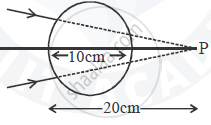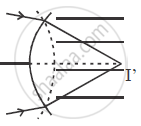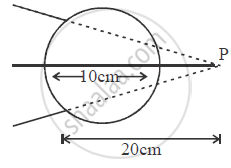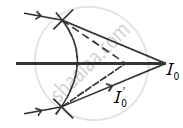Advertisement Remove all ads

# A Converging Beam of Light Travelling in Air Converges at a Point P as Shown in the Figure. When a Glass Sphere of Refractive Index 1 . 5 is Introduced in Between the Path of the Beam, - Physics

Numerical

A converging beam of light travelling in air converges at a point P as shown in the figure. When a glass sphere  of refractive index 1 . 5 is introduced in between the path of the beam, calculate the new position of the image. Also draw the ray diagram for the image formed.Advertisement Remove all ads

#### Solution

Case 1 : n1 = 1 n2 = 1.5

u = ∞1.5/V = 1/ ∞ = ((1.5 - 1))/5

1.5/V = 0.5/5

V = 15 cm

case 2 :1/V - 1.5/15 = (1-1.5)/-5

1/V = 0.5/5 + 1.5/15

1/V = 1/20

V = 20 cm

Concept: Refraction
Is there an error in this question or solution?
Advertisement Remove all ads

#### APPEARS IN

Advertisement Remove all ads

#### Video TutorialsVIEW ALL 

Advertisement Remove all ads
Share
Notifications

View all notifications

Forgot password?